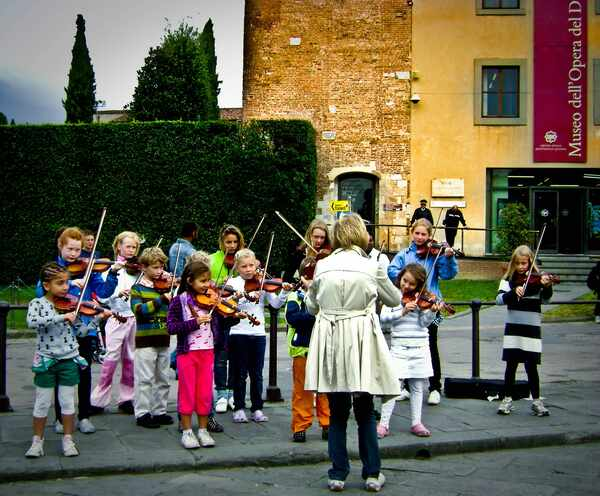# Math and Music: The Fascinating Intersection of Math and Musical Theory

The relationship between math and music has been observed throughout history, from the Pythagorean tuning system in ancient Greece to modern-day algorithmic composition. Whether it’s the rhythm of a beat or the harmonies of a melody, math is an essential component of music that has helped shape the sounds we hear today.

In this article, we’ll delve into the fascinating ways in which math and musical theory intersect. We’ll also look at how tutors can explore this connection, both within the traditional classroom setting and during online math classes.

## How Math and Music Connect

For centuries, the connection between math and music, two seemingly different disciplines, has been studied, celebrated, and even exploited. Let’s explore some of the ways they intersect:

### 1.   Rhythm and Timing

Music is all about rhythm and timing. Beats, tempo, and meter all rely on mathematical concepts such as division, ratios, and fractions. A simple example is the time signature, which is a fraction that represents the number of beats per measure. The most common time signature is 4/4, which means four beats per measure.

### 2.   Pitch and Harmony

Math is also essential in understanding pitch and harmony in music. The notes on a musical scale are based on a mathematical ratio of frequencies. The intervals between notes also follow mathematical ratios, such as the octave, which is a doubling of the frequency. Harmony, on the other hand, relies on the relationship between different pitches and the mathematical ratios between them.

### 3.   Musical Form and Structure

Music is not just a collection of sounds, but it has a structure and form. Mathematical concepts such as patterns, sequences, and symmetry play a vital role in creating musical forms. For example, the sonata form in classical music follows a specific structure of exposition, development, and recapitulation.

### 4.   Music Production

Math is also essential in music production, including recording and mixing. The digital audio workstation (DAW) software used by producers relies on mathematical algorithms for signal processing, compression, and equalization. Moreover, electronic music genres such as techno and EDM are often created using mathematical concepts such as algorithms and fractals.

### 5.   Music and Math Education

Finally, math and music are often intertwined in education. Learning music theory involves understanding mathematical concepts such as fractions, ratios, and geometry. Similarly, teaching math through music has been shown to be effective in engaging students and improving their mathematical skills.

## Different Ways to Teach Math Using Music

Since math and music have so much in common, can math tutors exploit these similarities to help kids learn more effectively? The answer is a resounding yes. You can teach core math topics like geometry, fractions, and counting using music.

Over the years, education experts have come up with different ways to teach math to students using music. Let’s explore some of the different ways you can do it:

### 1.   Math Songs

One of the easiest and most effective ways to teach math with music is by using math songs. You can find lots of educational songs online that teach everything from basic addition and subtraction to more complex topics like algebra and geometry. These songs are catchy and fun, which makes it easier for kids to remember math concepts.

### 2.   Rhythmic Counting

Another way to use music to teach math is through rhythmic counting. You can use a simple beat to help kids count numbers, skip count, or even learn fractions. For example, you can clap twice for every even number or clap three times for every multiple of three.

### 3.   Musical InstrumentsYou can also use musical instruments to teach math concepts. For example, you can use a xylophone to teach kids about patterns or a drum set to teach about fractions. You can also have kids create their own musical patterns using different instruments.

### 4.   Math-Based Dance

You can make math lessons more engaging by incorporating dance into the lesson. For example, you can have kids create a dance routine that includes counting, skip counting, or other math concepts. This is a great way to get kids up and moving while learning math.

### 5.   Musical Math Games

Finally, you can use musical math games. For example, you can play a game where kids have to identify the correct answer to a math problem based on the musical notes they hear. This is a fun way to reinforce math concepts and make learning more enjoyable.

## Final Thoughts

The intersection between math and music is a fascinating one and goes beyond the surface level. From rhythm and timing to music production, math plays an essential role in music theory and practice. This relationship has proved beneficial for math tutors who can use music as a tool to teach mathematical concepts to kids. Using music and math together, tutors can help make learning more enjoyable and effective.